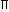# Aptitude - Volume and Surface Area - Discussion

### Discussion :: Volume and Surface Area - Data Sufficiency 1 (Q.No.1)

Each of the questions given below consists of a statement and / or a question and two statements numbered I and II given below it. You have to decide whether the data provided in the statement(s) is / are sufficient to answer the given question. Read the both statements and

• Give answer (A) if the data in Statement I alone are sufficient to answer the question, while the data in Statement II alone are not sufficient to answer the question.
• Give answer (B) if the data in Statement II alone are sufficient to answer the question, while the data in Statement I alone are not sufficient to answer the question.
• Give answer (C) if the data either in Statement I or in Statement II alone are sufficient to answer the question.
• Give answer (D) if the data even in both Statements I and II together are not sufficient to answer the question.
• Give answer(E) if the data in both Statements I and II together are necessary to answer the question.

1.

 What is the volume of 32 metre high cylindrical tank? I. The area of its base is 154 m2. II. The diameter of the base is 14 m.

 [A]. I alone sufficient while II alone not sufficient to answer [B]. II alone sufficient while I alone not sufficient to answer [C]. Either I or II alone sufficient to answer [D]. Both I and II are not sufficient to answer [E]. Both I and II are necessary to answer

Explanation:

Given, height = 32 m.

I gives, area of the base = 154 m2.Volume = (Area of the base x Height) = (154 x 32) m3.

Thus, I alone gives the answer.

II gives, radius of the base = 7 m.Volume =r2h =22 x 7 x 7 x 32m3 = 4928 m3. 7

Thus, II alone gives the answer.Correct answer is (C).

 Debanjan said: (Nov 9, 2011) We have to consider the thickness.

 Ganga Singh said: (Dec 12, 2016) Answer E is correct or not? If not then why?